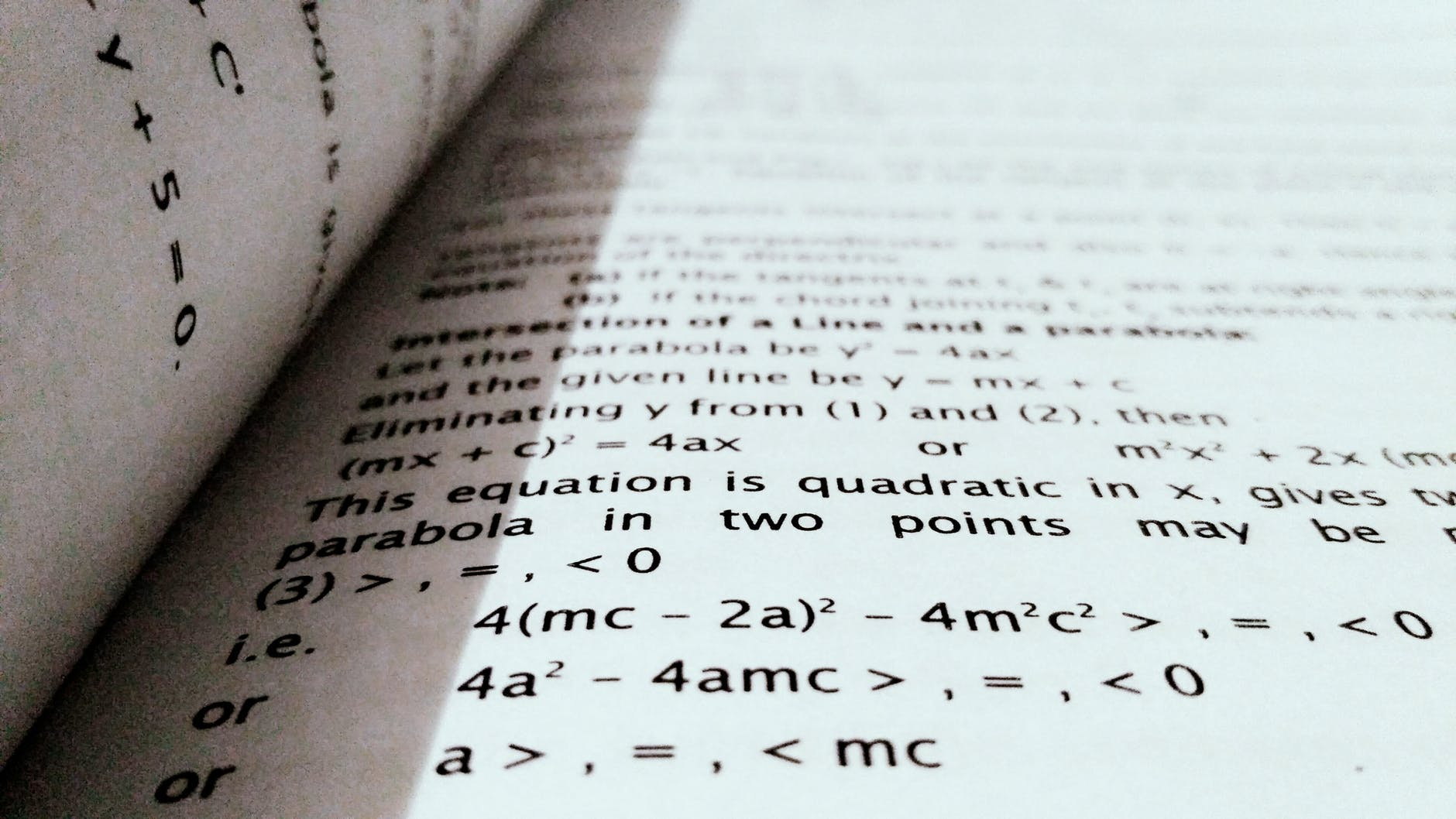# Using and Arrays and Functions in Excel VBA

In this post, we are going to look at using arrays and functions together. Our goal is to create a function that can divide the numbers in an array and count how many have a remainder.

Declare Function and Variables

First, we need to declare our function and variables. This is done in the code below.

``````Function remainderCount(rng as Range, n as integer) as Integer
Dim i as Integer, j as Integer
Dim nr As Integer, nc As Integer, c As Integer
nr=  rng.Rows.Count
nc = rng.Columns.Count``````

The name of our function is remainderCount. It takes to arguments. Rng is for the array and n is for the divisor. In the next to lines, we declare variables for the following.

• i for row
• j for column
• nr for number of rows
• nc for number of columns
• c for the number of numbers that have a remainder
• The last to lines are for counting the number of rows and columns

For Loop

Next, we need to create our nested for loop. This code has been used several times on this blog so we will not explain much here.

``````For i = 1 To nr
For j = 1 to nc
If rng.Cells(i,j) Mod n >= 1 Then
c = c+1
End If
Next J
Next i
remainderOne = c
End Function``````

The for loop goes first across columns and then down to the next row. In each cell, it checks to see that if the number in that cell is divided by our n if there is a remainder or not. If so then the c variable increase by 1. After this, the output of the function is set to whatever value c is,

Below is the full code followed by what this looks like in Excel.

``````Function remainderCount(rng as Range, n as integer) as Integer
Dim i as Integer, j as Integer
Dim nr As Integer, nc As Integer, c As Integer
nr=  rng.Rows.Count
nc = rng.Columns.Count
For i = 1 To nr
For j = 1 to nc
If rng.Cells(i,j) Mod n = 1 Then
c = c+1
End If
Next J
Next i
remainderCount = c
End Function``````

Below is the output

The answer is 4. This is because there are six numbers in the array. Two of the numbers are divisible by 4 (12 & 24) but the other four numbers are not (14, 18,19,27). The numbers that are not divisible by 4 make up the answer.

Conclusion

This is one of the more practical ways that you can employ knowledge of arrays with functions. Of course, there is an infinite number of ways to use these tools. This means that practice is the best way to learn how to apply these tools.